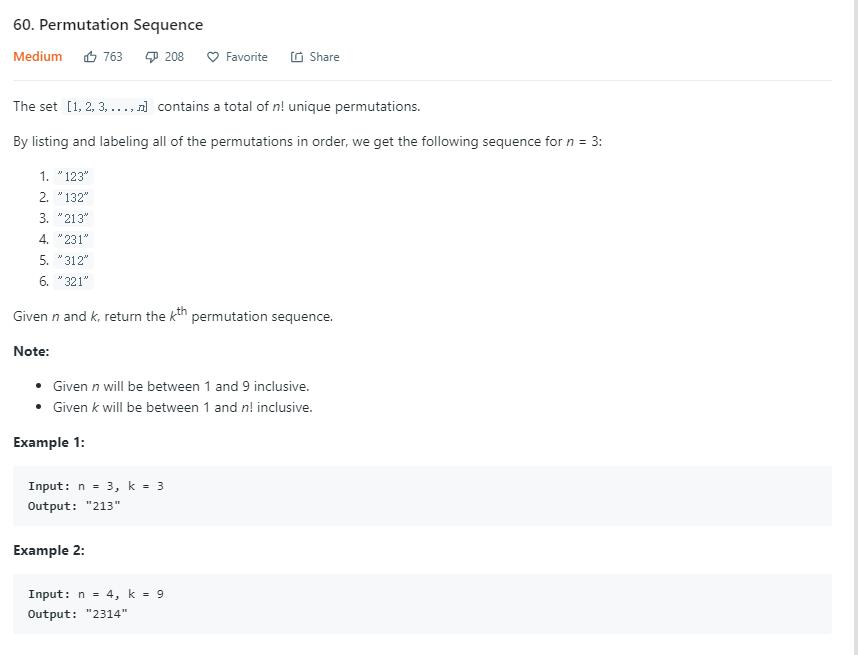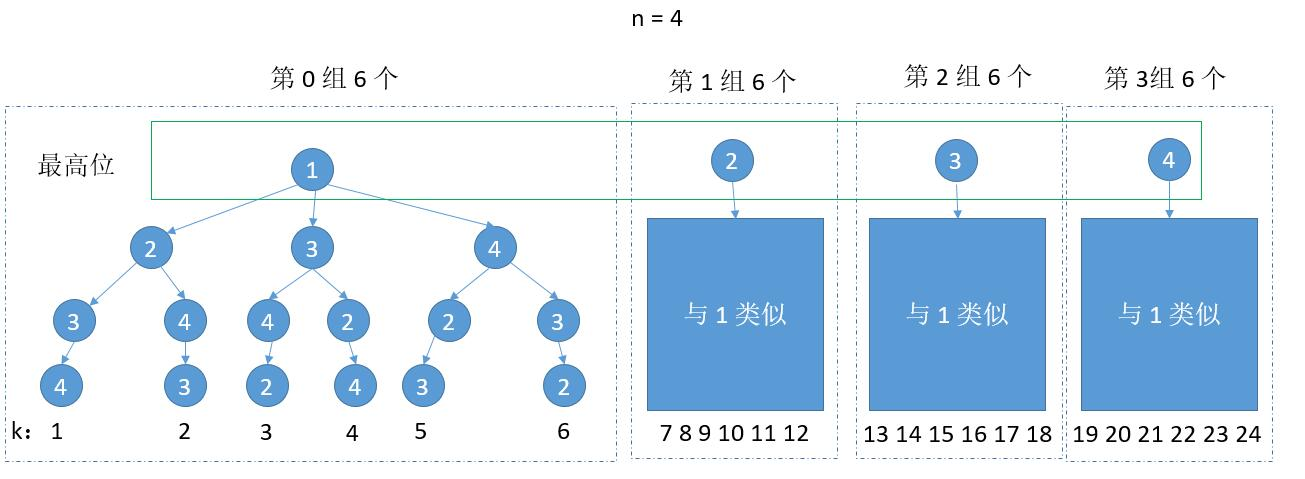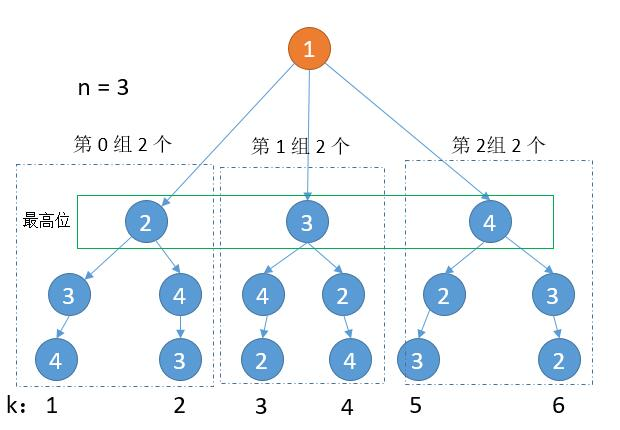# 题目描述（中等难度）# 解法一int perGroupNum = factorial(n - 1);
int groupNum = (k - 1) / perGroupNum;


k = k % perGroupNum;
k = k == 0 ? perGroupNum : k;


List<Integer> nums = new ArrayList<Integer>();
for (int i = 0; i < n; i++) {
}
int perGroupNum = factorial(n - 1);
int groupNum = (k - 1) / perGroupNum;
int num = nums.get(groupNum); //根据 groupNum 得到当前位
nums.remove(groupNum);//去掉当前数字public String getPermutation(int n, int k) {
List<Integer> nums = new ArrayList<Integer>();
for (int i = 0; i < n; i++) {
}
return getAns(nums, n, k);
}

private String getAns(List<Integer> nums, int n, int k) {
if (n == 1) {
//把剩下的最后一个数字返回就可以了
return nums.get(0) + "";
}
int perGroupNum = factorial(n - 1); //每组的个数
int groupNum = (k - 1) / perGroupNum;
int num = nums.get(groupNum);
nums.remove(groupNum);
k = k % perGroupNum; //更新下次的 k
k = k == 0 ? perGroupNum : k;
return num + getAns(nums, n - 1, k);
}
public int factorial(int number) {
if (number <= 1)
return 1;
else
return number * factorial(number - 1);
}


k = k % perGroupNum; //更新下次的 k
k = k == 0 ? perGroupNum : k;


public String getPermutation(int n, int k) {
List<Integer> nums = new ArrayList<Integer>();
int factorial = 1;
for (int i = 0; i < n; i++) {
if (i != 0) {
factorial *= i;
}
}
factorial *= n; //先求出 n 的阶乘
StringBuilder ans = new StringBuilder();
k = k - 1; // k 变为 k - 1
for (int i = n; i > 0; i--) {
factorial /= (nums.size()); //更新为 n - 1 的阶乘
int groupNum = k / factorial;
int num = nums.get(groupNum);
nums.remove(groupNum);
k = k % factorial;
ans.append(num);

}
return ans.toString();
}